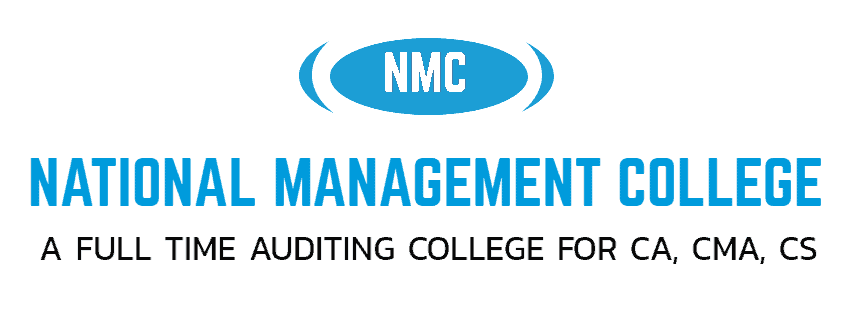# CA Foundation (Paper 3) Business Maths and Statistics 7

NATIONAL MANAGEMENT COLLEGE, THUDUPATHI.

CA FOUNDATION

PAPER – 3: BUSINESS MATHEMATICS, LOGICAL REASONING AND STATISTICS

Revision test 7 (02.04.2022)

Time Allowed : ½ hour                                               Maximum Marks: 25

1)Find the value of  dy /dx  if

(a) log e  (b) 1+logx (c) y logx  (d) none of these

2) If   f(x) = a (x2 + x +1)2   and  f ‘ (–1) = – 6  then the value of  a =

(a) 1  (b) 2 (c) 3  (d) 4

3)If  2x -2y = 2 x+y , then dy/dx  at x = y =2

1. a) 1 b) 2 c) 4 d) 5

4) If the Cost of function of a commodity is given by  , where C stands for cost and  stands for output. If the average cost is equal to the marginal cost then the output = _____

(a) 5  (b) 10  (c) 15  (d) 20

5)  If     then dy/dx is equal to :

1. a) b) c)d)

6)  The speed of a train at a distance x ( from the starting point ) is given by  . what  is the rate of change ( of distance)  at  ?

1. a) -1 b)0 c) 1 d) 2

7) U  at   find dU/dt

a)-11 b)11 c)-16 d)16

8) The gradient of the curve  at  is

1. a) -12 b) 12 c) 0 d) 1

9) Find slope of tangent of curve Y =   at

a)3/16 b)5/17 c) 9/11 d)None of these

10) If y =  , then   +   –    yields,

1. a) 3 b)  1 c) 0 d) 4

11) . If f(x) = xk and f `(1) = 10 then the value of k is

a)10 b) -10 c) 1/10 d) none of these

12) Given x = 2t + 5; y = t2-2, then dy/dx  is calculated as:

1. a) t b) 1/t c) -1/t d) none of these

13)  If   +   = 0 , then     =

1. a) 0 b) 1 c)-1 d) 2

14) The cost of C of a product is a function of the quantity x of the product:                         C(x) = x2 – 400x + 50. Find the quantity for which the cost is minimum.

1. a) 1000 b)1500 c)2000 d) 3000

15)I f u = xm yn  then

1. a) du = mxm-1yn+ nxmyn-1 b)
2. c) d)

16) The maximum value of  is

1. a) b) c) d)none of these

17) The slope of the tangent to the curve   , where the line  y=2 cuts the curve in the first quadrant is  ,

1. a) 2 b)  3 c) -3 d) -2

18) If   being “a” constant then dy/dx is

1. a)
2. b)

c)

1. d) none

19) The derivative of a function   is

1. a)   b) 1+                   c)       d)none of these

20)Find    , if  Y =   ,

1. a) b) c) d)

21)   then  =

1. a) b) c) d)

22)  =

1. a) 1                           b) constant             c)  0                         d)none of these

23) A company can produce a maximum of 1500 widgets in a year. If they sell x  widgets during the year then their profit, in dollars, is given by, P(x)=30,000,000−360,000x+750x2−13×3           How many widgets should they try to sell in order to maximize their profit?

1. a) 0 b) 300 c) 1200 d) 1500

24) The production costs, in dollars, per week of producing x widgets is given by, C(x)=4000−32x+0.08x2+0.00006x3   and the demand function for the widgets is given by,   D(x)=250+0.02x−0.001x2  What is the  marginal revenue  when x=200 and x=400?

a)152, 312 b) 132,-218 c) 164, -245 d) 112,156

25) The slope of the tangent at the point (2,-2) to the curve   is given by

a)0 b) 1 c) -1 d) none